e-ISSN: 2319-9849

All submissions of the EM system will be redirected to Online Manuscript Submission System. Authors are requested to submit articles directly to Online Manuscript Submission System of respective journal.

# Thermodynamics of Solvation and Apparent Molar Volumes for O-Toluic Acid in Ethanol-Water Mixtures At 298.15K

Esam A Gomaa1*, Elsayed M Abou Elleef2, Kamal M Ibrahim1, Amr A Ibrahim1 and Maii S Mashaly1

1Chemistry Department, Faculty of Science, Mansoura University, 35516-Mansoura, Egypt

2Basic Science Department, Delta Higher Institute for Engineering & Technology, 35681- Dalkha, Mansoura, Egypt

*Corresponding Author:
Esam A Gomaa
Chemistry Department, Faculty of Science
Mansoura University, 35516-Mansoura, Egypt

Received date: 17/05/2014; Revised date: 15/06/2014; Accepted date: 24/06/2014

Visit for more related articles at Research & Reviews: Journal of Chemistry

## Abstract

The solubilities of o-toluic acid have been determined in water and ethanol-water mixtures at different temperatures. From the solubility data, the values of the solubility product of o-toluic acid were calculated, and from these values, Gibbs energy for the solution processes was determined. The respective thermodynamic functions for mixing and solvation processes as well as the activity coefficients for the solute were calculated. In addition, the thermodynamic quantities for the transfer process of o-toluic acid from water to ethanol-water mixtures were also calculated in order to estimate the non-ideality due to the mean activity coefficient of ions, contributions of hydrogen-bonds or of other dipolar interactions. The molar, Van der Waals, electrostriction and apparent molar volumes of o-toluic acid in various solvents at 298.15K are also calculated. The results are discussed on the basis of transfer functions and in terms of solute- solute and solute-solvent interactions.

#### Keywords

o-toluic acid, mixed solvents, solubility, thermodynamics, and transfer.

#### Introduction

Solubility of solid compounds in solvents is one of the most fundamental physicochemical properties, particularly useful in a wide variety of phenomena relevant to biological, pharmaceutical, environmental and other organic, physical and analytical chemistry, and engineering sciences. Tools that can quickly estimate the solubility as a function of temperature and solvent composition are considered as being crucial in the engineering practice of today.

Carboxylic acids are widely used as intermediates in large scale preparations of resins, plasticizers, dyes, inks and adhesives, pharmaceuticals as well as in the food industry [1-4]. They are also of interest in environmental chemistry, as they might occur as oxidation residues in the polyester industry, or they appear as metabolites. Carboxylic acids are also of biological importance and represent a particularly useful series of model compounds for studying hydrogen bonding and hydrophobic interactions [5-8]. As a consequence of their widespread use and importance, solubilities of carboxylic acids as a function of temperature might be considered being extensively measured, recommending them as test compounds for predictive methods, and yet, at times, discrepancies in published values, concerning accuracy and reproducibility of the gathered data, may occur.

The aim of this paper is to present new Experimental solubility data for o-toluic acid dissolved in ethanol-water mixtures with different concentrations of components.

#### Experimental

Chemicals and Reagents

O-toluic acid and ethanol were purchased from Merck Company with high degree of purity and used without further purification.

Preparation of mixed solvent and saturated solutions

The mixed solvent, (ethanol + water by value percent of ethanol = 0, 20, 40, 60, 80 and 100% by volume), were prepared from deionized water and fairly pure ethanol. Then, saturated solutions of o-toluic acid were prepared by dissolving different amounts in closed test tubes containing different (ethanol + water) mixtures. These mixtures were then saturated with nitrogen gas an inert atmosphere. The tubes were placed in a shaking thermostat (Model Gel) for a period of four days till equilibrium reached. The solubility, S (mole/Kg.solvent), of o-toluic acid in the mixed solvent at 298.15K was determined gravimetrically by the solvent evaporating method. All the solubility experiments were repeated at least three times and the results were averaged.

#### Results and Discussion

We may consider the following equilibrium in its saturated solution at a given constant temperature.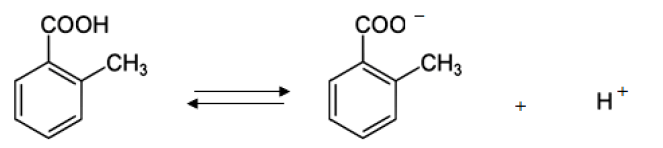The solubility product constant (Ksp) is given by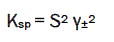(1)

where S is the molal solubility and γ± is the mean molal activity coefficient.

The activity coefficient may be determined using the extend Debye-Hückel law :(2)

Where Z+ and Z- are the charges of ions in solutions, A= 1.823x106(ε.T)-3/2 , B = 50.29 (ε.T), and r° is the solvated radius, the ionic strength I, defined as,(zi is the charge on ion i, and mi is the molality of ion i) and the ionic strength, I emphasizes the charges of ions because the charge numbers occur as their squares.

The solubility product was calculated by the use of equation (3).(3)

The molal solubility, activity coefficient, ionic strength and solubility product data are given in Table (1), the Gibbs free energies of solvation and the transfer Gibbs free energies from water to mixed solvents were calculated by using equations (4) and (5) [10-73]. Their values are tabulated also in Table (1).

Table 1: Molal solubility, ionic strength, activity coefficient, solubility product, Gibbs free energies change and transfer Gibbs free energies for o-toluic in mixed EtOH -H2O solvents at 298 K.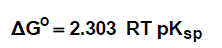(4)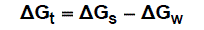(5)

Where (s), (w) denote solvent and water, respectively.

The molal solubility of saturated solution of o-toluic acid in mixed solvents found to increase with the increase the mole fraction of EtOH in the mixed solvent (see Fig. 1) while activity coefficient found to decrease (see Fig. 2). The Gibbs free energies of solvation of o-toluic acid in mixed solvents found to decrease with the increase the mole fraction of EtOH in the mixed solvent and the transfer Gibbs free energies from water to mixed solvents found to increase with negative value (see Fig. 3&4). These indicate that, adding more organic solvent to the mixture, significant changes in acid solubility in mixtures of ethanol+ water were verified. Therefore, the addition of an organic solvent to water favored the solubilisation of benzoic acid.

Figure 1: Relation between molal solubility (S) of o-toluic acid and mole fraction (xs) of ethanol in mixed EtOH -H2O solvents at 298 K.

Figure 2: Relation between activity coefficient (γ ±) of o-toluic acid and mole fraction (xs) of ethanol in mixed EtOH -H2O solvents at 298 K.

Figure 3: Relation between change in free energy of solvation (ΔGs ) of o-toluic acid and mole fraction (xs) of ethanol in mixed EtOH -H2O solvents at 298 K.

Figure 4: Relation between change in free energy of transfer (ΔGt ) of o-toluic acid and mole fraction (xs) of ethanol in mixed EtOH -H2O solvents at 298 K.

The molar volumes (VM) of o-toluic acid were obtained by dividing the molar mass by the densities and their values are listed in Table 2. The packing density as reported by Gomaa and Kim [34-61], i.e. the relation between the Van der Waals volume (VM) and the molar volume (VM) of relatively large molecules (above 40) was found to be a constant value and equal to 0.661.

Table 2: Molar volumes (VM), Van der Waals volumes (VW), electrostriction volumes (Ve) and apparent molar volumes (VΦ) for saturated solutions of o-toluic acid in mixed EtOH -H2O solvents at 298 K. (in cm3mole-1).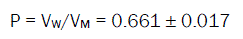(6)

The electrostriction volume (Ve), which is the volume compressed by the solvent, was calculated using the following equation [18-32,70]: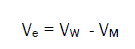(7)

The molar, Van der Waals and electrostriction volumes of o-toluic acid in various solvents at 298.15K are tabulated in Table 2.The apparent molar volumes, VΦ,  were calculated using the following equation [71,72]:(8)

where M is the molar mass of o-toluic acid, m is the concentration, d and do are the densities of saturated solution and pure solvents, respectively. The values of VΦ for o-toluic acid in various solvents at 298.15K are presented in Table 2.

The data in Table 2 shows an increase in the values of VM and VM and decrease at the values of the third Ve. A negative increase of electrostriction values was observed for o-toluic acid solutions by increasing the proportions of ethanol in the mixtures. It was observed from the different volume values, that all volumes for o-toluic acid increased by increasing ethanol content in the mixed solvent due mainly to the higher solvation. Also the electrostriction volumes increase in negativity confirming the increase in solvent effect by more adding ethanol to the mixtures.

#### Conclusions

The solubility of saturated solution of o-toluic acid in mixed solvents found to increase with the increase the mole fraction of EtOH in the mixed solvent. In addition, Gibbs energy change for the solution processes and free energies of transfer were also calculated in order to estimate the contributions solutesolvent interactions related ion association is based on changes in the electrostatic properties of the solvent, solute and ion solvation as well as on the ionic strength of the medium. The saturated solution of o-toluic acid in the mixed solvent is perfectly non-ideal. The non-ideality is partly due to the mean activity coefficient of ions in the solution and partly due to the ion association phenomenon. It was observed from the different volume values, that all volumes for o-toluic acid increased by increasing ethanol content in the mixed solvent due mainly to the higher solvation. Also the electrostriction volumes increase in negativity confirming the increase in solvent effect by more adding ethanol to the mixtures.

#### Acknowledgment

This work was supported by Chemistry Department, Faculty of Science, Mansoura University and Basic Science Department, Delta Higher Institute for Engineering and Technology, Dakhlia, Mansoura, Egypt.

Replica watches